﻿ Introduction to Logarithmic Functions
INTRODUCTION TO LOGARITHMIC FUNCTIONS

In this section we continue to explore logarithms, but now with an emphasis on the family of logarithmic functions.

DEFINITION logarithmic function
A logarithmic function is a function of the form $\,y=\log_b\,x\,$,
where $\,b\,$ is a positive number not equal to $\,1\,$, and $\,x\gt 0\,$.

The number $\,b\,$ is called the base of the logarithm.

When the base is $\,\text{e}\,$, the function is called the natural logarithm,
and can be written as $\,y=\ln\,x\,$.
(Note: $\,\text{e}\approx 2.71828\,$)

When the base is $\,10\,$, the function is called the common logarithm,
and can be written as $\,y=\log\,x\,$.
Be Alert to Differences in Notation

Some academic disciplines use the natural log a lot, but the common log not so much.
These disciplines often use $\,\log\,$ to mean log base e, instead of log base ten.
Be sure to check what notation is being used whenever you start communicating with someone new.

Furthermore, some mathematicians (this author included) tend to call more general functions
where a logarithm is acting on variable input a logarithmic function;
say, $\,y=\ln (2x-3)\,$ or $\,y=-7\log(5-x)\,$.
For the purposes of this section, however,
the phrase logarithmic function refers only to functions of the form $\,y=\log_b\,x\,$.

There are two basic shapes to the graphs of logarithmic functions,
depending on whether the base is greater than $\,1\,$, or between $\,0\,$ and $\,1\,$:

 $\,y=\log_b\,x\,$   for   $\,b\gt 1\,$ $\,y=\log_b\,x\,$   for   $\,0\lt b\lt 1\,$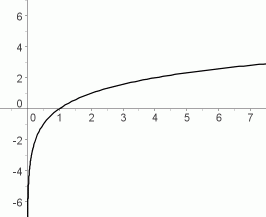increasing functions decreasing functions
 PROPERTIES OF THE GRAPH for $\,b\gt 1\,$ An increasing function has the following property: as you walk along the graph, going from left to right, you are always going UPHILL. The following are equivalent for a function $\,f(x)=\log_b\,x\,$: $\,f\,$ is an increasing function $\,b\gt 1\,$ For increasing logarithmic functions: as $\,x\rightarrow\infty\,$, $\,y\rightarrow\infty\,$ Read this aloud as:   as $\,x\,$ goes to infinity, $\,y\,$ goes to infinity This is the right-hand end behavior: as we move farther and farther to the right on the graph, the $\,y$-values of the points are increasing, without bound. For example, want to get to a point on the graph that is (say) one million units above the $\,x$-axis? You can do it—but you'll have to go a lloonngg way to the right, because the function increases very slowly. as $\,x\rightarrow 0^+\,$, $\,y\rightarrow -\infty\,$ Read this aloud as:   as $\,x\,$ approaches zero from the right-hand side, $\,y\,$ goes to negative infinity For additional practice with sentences like   ‘$\,x\rightarrow\infty\,$, $\,y\rightarrow\infty\,$’   and ‘as $\,x\rightarrow 0^+\,$, $\,y\rightarrow -\infty\,$’, you may want to review Basic Models You Must Know.$y = \log_b\,x\,$   for   $\,b\gt 1$ increasing functions as $\,x\rightarrow\infty\,$, $\,y\rightarrow\infty\,$ as $\,x\rightarrow 0^+\,$, $\,y\rightarrow -\infty\,$
 PROPERTIES OF THE GRAPH for $\,0 \lt b\lt 1\,$ A decreasing function has the following property: as you walk along the graph, going from left to right, you are always going DOWNHILL. The following are equivalent for a function $\,f(x)=\log_b\,x\,$: $\,f\,$ is a decreasing function $\,b\,$ is between $\,0\,$ and $\,1\,$ For decreasing logarithmic functions: as $\,x\rightarrow\infty\,$, $\,y\rightarrow -\infty\,$ Read this aloud as:   as $\,x\,$ goes to infinity, $\,y\,$ goes to negative infinity as $\,x\rightarrow 0^+\,$, $\,y\rightarrow \infty\,$ Read this aloud as:   as $\,x\,$ approaches zero from the right-hand side, $\,y\,$ goes to infinity$y = \log_b\,x\,$   for   $\,0 \lt b\lt 1$ decreasing functions as $\,x\rightarrow\infty\,$, $\,y\rightarrow -\infty\,$ as $\,x\rightarrow 0^+\,$, $\,y\rightarrow \infty\,$
Properties that All Logarithmic Functions Share

Let $\,f(x)=\log_b\,x\,$, where $\,b\,$ is a positive number not equal to $\,1\,$, and $\,x\gt 0\,$.
For all (allowable) bases $\,b\,$, logarithmic functions share the following properties:

 THE DOMAIN IS THE SET OF POSITIVE NUMBERS: $\text{dom}(f) = (0,\infty)$ If the graph of a logarithmic function is ‘collapsed’ into the $\,x$-axis, sending each point on the graph to its $\,x$-value, then all positive $\,x$-values will be hit. Logarithms only know how to act on positive inputs. For basic information on the domain and range of a function, you may want to review: Domain and Range of a Function Having trouble understanding the expression ‘$\,(0,\infty)\,$’? Then, you may want to review Interval and List Notation.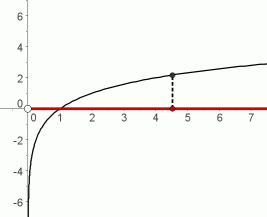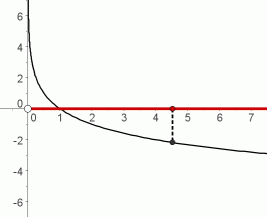THE RANGE IS THE SET OF ALL REAL NUMBERS: $\text{ran}(f)=\mathbb{R}$ If the graph of a logarithmic function is ‘collapsed’ into the $\,y$-axis, sending each point on the graph to its $\,y$-value, then all $\,y$-values will be hit. In particular, even though increasing logarithm curves rise very slowly for large inputs, they WILL eventually reach any desired output, no matter how big (and positive) it may be! And, even though decreasing logarithm curves fall very slowly for large inputs, they WILL eventually reach any desired output, no matter how big (and negative) it may be! Indeed, the fact that logarithmic functions increase/decrease very slowly for large inputs is an important feature of their graphs, which makes them particularly valuable in modeling slowly-changing behavior.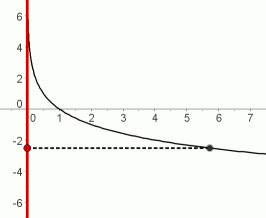THE GRAPH CROSSES THE $\,x\,$-AXIS AT $\,x=1\,$ For allowable values of $\,b\,$: $$\log_b\,1 \overset{\text{always}}{\ \ \ =\ \ \ } 0\ , \ \ \ \text{ since }\ \ \ b^0 \overset{\text{always}}{\ \ \ =\ \ \ } 1$$ So, when the input is $\,1\,$ to the function $\,\log_b\,$, the output is $\,0\,$. Thus, the point $\,(1,0)\,$ lies on the graph of every logarithmic function.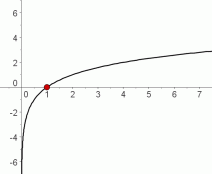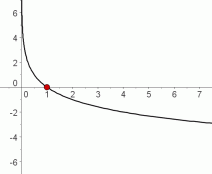THE GRAPH PASSES BOTH THE VERTICAL AND HORIZONTAL LINE TEST
VERTICAL LINE TEST:

Imagine a vertical line sweeping through a graph, checking each allowable $\,x$-value:
if it never hits the graph at more than one point, then the graph is said to pass the vertical line test.
All functions pass the vertical line test, since the function property is that each input has exactly one output.

 passes the vertical line test: each $\,x$-value has only one $\,y$-value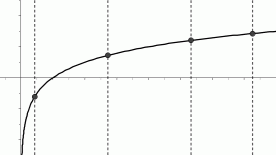all functions pass the vertical line test fails the vertical line test: there exists an x-value that has more than one y-value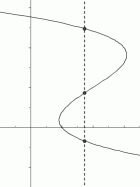HORIZONTAL LINE TEST:

Imagine a horizontal line sweeping through a graph, checking each allowable $\,y$-value:
if it never hits the graph at more than one point, then the graph is said to pass the horizontal line test.
Some functions pass the horizontal line test, and some do not.

 passes the horizontal line test: each $\,y$-value has only one $\,x$-value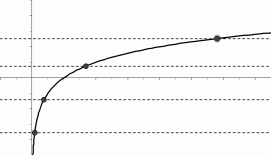all logarithmic functions pass the horizontal line test fails the horizontal line test: there exists a $\,y$-value that has more than one $\,x$-value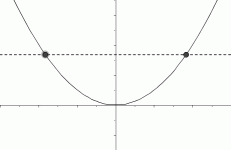some functions fail the horizontal line test

Thus, logarithmic functions have a wonderful property:
each input has exactly one output (passes the vertical line test), and
each output has exactly one input (passes the horizontal line test).

For such functions, you can think of the inputs/outputs as being connected with strings:
pick up any input, and follow its ‘string’ to the unique corresponding output;
pick up any output, and follow its ’string’ to the unique corresponding input.
That is, there is a one-to-one correspondence between the inputs and outputs.
Functions with this property are called one-to-one functions.

Master the ideas from this section